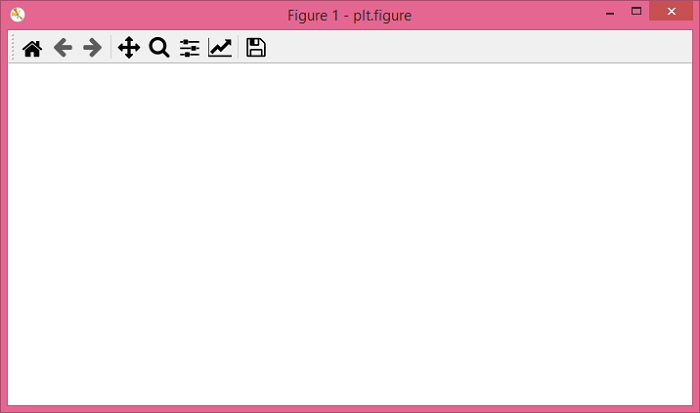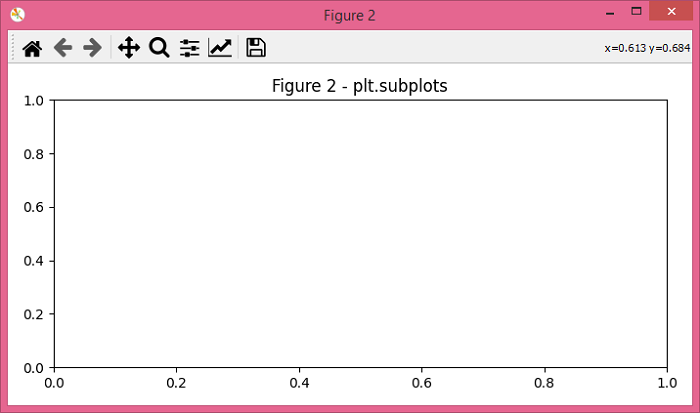# Matplotlib â€“ Difference between plt.subplots() and plt.figure()

plt.figure() − Creates a new figure or activates an existing figure.

plt.subplots() − Creates a figure and a set of subplots.

Let's take an example to understand the difference between plt.subplots() and plt.figure().

## Steps

• Set the figure size and adjust the padding between and around the subplots.

• Create a new figure or activate an existing figure. Use plt.figure() method.

• Create a figure and a set of subplots. Use plt.subplots() method.

• To display the figure, use Show() method.

## Example

from matplotlib import pyplot as plt

# Set the figure size
plt.rcParams["figure.figsize"] = [7.00, 3.50]
plt.rcParams["figure.autolayout"] = True

# Create a new figure using plt.figure
fig1 = plt.figure("Figure 1 - plt.figure")

# Create a figure and set of subplots using plt.subplots
fig2, ax = plt.subplots()
plt.title("Figure 2 - plt.subplots")

# Display the plot
plt.show()


## Output

plt.figure() produces the following OutputAnd, plt.subplots() produces the following Output −Updated on: 11-Oct-2021

823 Views